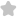Which of the following given option...
Question# Which of the following given options is/are incorrect? If p(x)=9(x) g(x) +r(x) (By Division Algorithm) where p(x), g(x) are any two polynomials with g(x) + 0, then (a) r(x)=0 always (b) degree of r(x) < degree of g(x) always (c) either r(x)= 0 or degree of r(x) IIT/JEE Maths SolutionShare1894.0 (1 ratings)( (a, b, d) ) (a) If ( p(x) ) is not divisible by ( g(x), ) then ( r(x) eq 0 therefore(a) ) is not true (b) If ( p(x) ) is divisible by ( g(x) ), then ( r(x)=0 ) for all ( x ) i.e., ( r(x) ) is a zero polynomial whose degree is not defined. ( therefore(b) ) is not true (c) is clearly true [ : division algorithm rule] (d) since degree of ( r(x)< ) degree of ( g(x) ) or ( r(x)=0, ) but ( g(x) eq 0 ) ( therefore r(x)=g(x) ) is not true.1894.0 (1 ratings) Rate SolutionShareQuick and Stepwise Solutions Just click and SendOVER 20 LAKH QUESTIONS ANSWERED Download App for Free